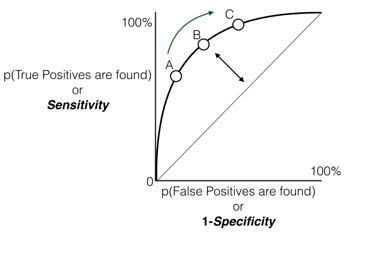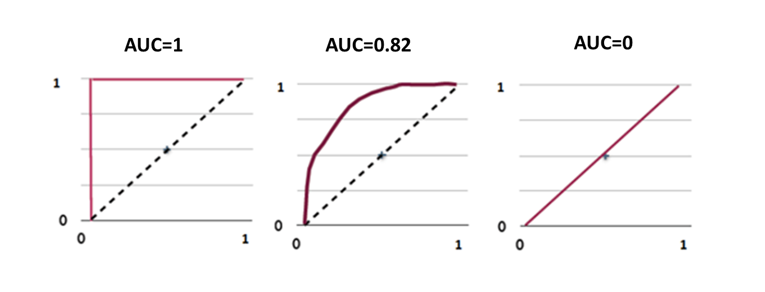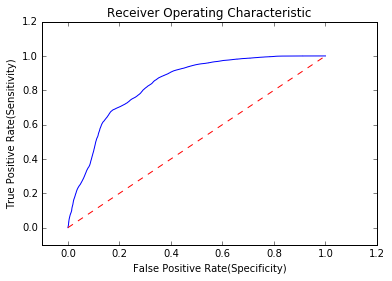• No products in the cart.

204.4.4 ROC and AUC

What they are and why they are important.
Link to the previous post : https://statinfer.com/204-4-3-more-on-sensitivity-and-specificity/

ROC Curve

• If we consider all the possible threshold values and the corresponding specificity and sensitivity rate what will be the final model accuracy.
• ROC(Receiver operating characteristic) curve is drawn by taking False positive rate on X-axis and True positive rate on Y- axis.
• ROC tells us, how many mistakes are we making to identify all the positives?ROC Curve – Interpretation

• How many mistakes are we making to identify all the positives?
• How many mistakes are we making to identify 70%, 80% and 90% of positives?
• 1-Specificty(false positive rate) gives us an idea on mistakes that we are making
• We would like to make 0% mistakes for identifying 100% positives
• We would like to make very minimal mistakes for identifying maximum positives
• We want that curve to be far away from straight line
• Ideally we want the area under the curve as high as possible

ROC and AUC

• We want that curve to be far away from the straight line. Ideally, we want the area under the curve as high as possible.
• ROC comes with a connected topic, AUC. Area Under the Curve.
• ROC Curve Gives us an idea on the performance of the model under all possible values of threshold.
• We want to make almost 0% mistakes while identifying all the positives, which means we want to see AUC value near to 1.

AUC

• AUC is near to 1 for a good modelROC and AUC Calculation

Building a Logistic Regression Model

In :
###for visualising the plots use matplotlib and import roc_curve,auc from sklearn.metrics
from sklearn.metrics import roc_curve, auc
import matplotlib.pyplot as plt
%matplotlib inline

actual = Fiber_df[['active_cust']]
false_positive_rate, true_positive_rate, thresholds = roc_curve(actual, predicted_values1)
plt.plot(false_positive_rate, true_positive_rate)
plt.plot([0,1],[0,1],'r--')
plt.xlim([-0.1,1.2])
plt.ylim([-0.1,1.2])
plt.ylabel('True Positive Rate(Sensitivity)')
plt.xlabel('False Positive Rate(Specificity)')
plt.show()In :
###Threshold values used for the roc_curve can be viewed from threshold array
thresholds
Out:
array([  2.00000000e+00,   1.00000000e+00,   9.99978894e-01, ...,
8.28263852e-03,   8.28015047e-03,   9.42770507e-04])
In :
###Area under Curve-AUC
roc_auc = auc(false_positive_rate, true_positive_rate)
roc_auc
Out:
0.83503740455417319

The next post is about what is a best model.
Link to the next post : https://statinfer.com/204-4-5-what-is-a-best-model/
21st June 2017
0 responses on "204.4.4 ROC and AUC"